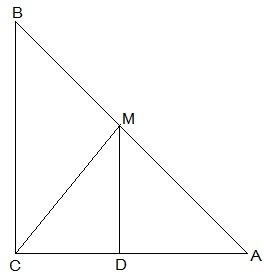Guru

# ABC is a triangle right angled at C. A line through the mid-point M of hypotenuse AB and parallel to BC intersects AC at D. Show that(iii) CM = MA = ½ AB Q.7(3)

• 0

what is the tricky way for solving the question of class 9th ncert math of Quadrilaterals chapter of ncert of exercise 8.2 of math give me the best and simple way for solving this question in easy and simple way ABC is a triangle right angled at C. A line through the mid-point M of hypotenuse AB and parallel to BC intersects AC at D. Show that(iii) CM = MA = ½ AB

Share

1. :(iii) In ΔAMD and ΔCMD,

AD = CD (D is the midpoint of side AC)